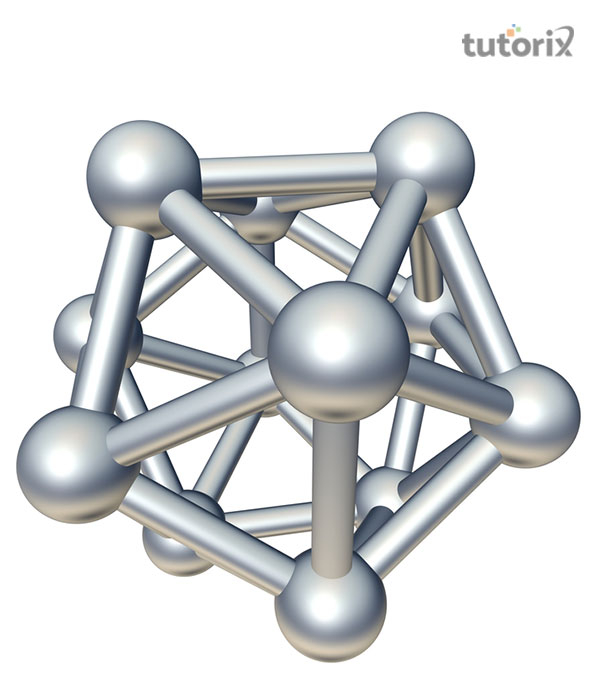# Boron

## Introduction

The elements of Group 13 (III A) are boron (B), aluminium (Al), gallium (Ga), indium (In), and thallium (Th) (Tl). Due to the existence of three valence electrons, these elements are also known as earth metals and triels. The outer shell electronic configuration of Boron can be written as $\mathrm{1s^2\:2s^2\:2p^1}$. In the following sections we would discuss the occurrence, physical and chemical properties of Boron.

## Occurrence and Extraction of Boron

Boron is a relatively uncommon element that makes up roughly 9 parts per million (ppm) of the earth's crust. Davy initially isolated the element in 1807 by fusing boric acid with potassium. It is mostly found in the form of concentrated deposits of borax $\mathrm{(Na_2B_4O_7.10H_2O)}$ and boric acid $\mathrm{(H_3BO_3)}$ and does not occur in the free state. Boranite $\mathrm{(2Mg_3B_3O_{15}.MgCl_{2})}$, Colemanite $\mathrm{(Ca_2B_6O_{11}.5H_2O)}$, boranatrocalcite $\mathrm{(Ca_2B_4O_7.NaBO_2.8H_2O)}$, and Kernite $\mathrm{(Na_2B_4O_7.4H_2O)}$ are some additional notable minerals.

## Physical Properties of Boron

### Ionisation Energy

Because the p-electrons are less tightly bound than the s-electrons, the first IE of Group IIIA elements is lower than that of Group 2 elements. The values of the first three ionisation energies for Boron is shown in Table. In comparison to the first IE, the second and third IEs are higher. Boron, as predicted, has the greatest IE value.

Element $\mathrm{IE_1}$ $\mathrm{IE_2}$ $\mathrm{IE_3}$ $\mathrm{IE_1 + IE_2 + 1E_3}$
Boron 801 2427 3659 6887

### Oxidation State

Boron's oxidation state is (+III) in all of its compounds. Other elements have an oxidation state of (+I). The stability of (+III) states reduces as one moves down the group, whereas (+I) state stability improves.

### Nature of Compounds

Because to its small ionic size and high IE, boron produces highly covalent compounds. Owing to the same reason Boron is a typical non-metal.

## Structure, Melting Point and Boiling Point

Boron has an interesting icosahedral structure, with $\mathrm{B_{12}}$ as the basic unit. Except for Ga, the other elements have a metal structure that is closely packed. As a result, the melting and boiling temperatures of B are quite high.Figure 1: Icosahedral B12 Unit

## Diagonal Relationship Between Boron and Silicon

• Allotropy: Both amorphous and crystalline forms occur for these elements.

• Occurrence: Both of these elements are only found in their combined condition in nature. They do not occur freely.

• Metallic Character: Because of their high IE, both of these elements are non-metals and insulators.

• Densities and Melting Point: Low densities and high melting points characterise these elements.

• Nature of Compounds: They form covalent complexes because to their high IE.

• Oxides and Oxoacids: Both of these elements burn in air to generate oxides that are sparingly soluble but have a high melting point. The oxides are extremely stable and only slightly acidic. When the oxides dissolve in water, they produce weak acids.

$$\mathrm{H_3BO_3 + 2H_2O\:\rightarrow\:H_3O^+ + [B(OH)_4]^–}$$

$$\mathrm{H_4SiO_4 + 2H_2O\:\rightarrow\:H_3O^+ + [Si(OH)_5]^–}$$

## Chemical Properties of Boron

### Reaction with Air and Water

Boron is highly unreactive in its pure crystalline state, but it is quite reactive in its finely split amorphous state. It produces $\mathrm{B_2O_3}$ when heated in air, and BN is created at extremely high temperatures. With $\mathrm{H_2O}$, it does not react.

$$\mathrm{4B+3O_2\:\rightarrow\:2B_2O_3}$$

$$\mathrm{2B + N_2\:\rightarrow\:2BN}$$

### Reaction with Acid and Bases

Hot concentrated sulphuric and nitric acids are reduced by amorphous boron.

$$\mathrm{B + 3HNO_3\:\rightarrow\:H_3BO_3 + 3NO}$$

$$\mathrm{2B + 3H_2SO_4 \:\rightarrow\:2H_3BO_3 + 3SO_2}$$

Boron liberates $\mathrm{H_2}$ from fused caustic alkalis, resulting in the formation of borates

$$\mathrm{2B + 6NaOH\:\rightarrow\:2Na_3BO_3 + 3H_2}$$

### Reaction with Ammonia

Except for boron, which reacts with $\mathrm{NH_3}$ at extremely high temperatures to form nitride, all metals react with $\mathrm{NH_3}$ to form amides.

$$\mathrm{2B + 2NH_3\:\rightarrow\:2BN + 3H_2}$$

## Uses of Boron

• Boron is utilised in the production of high-impact steel.

• Boron is employed as a flux in silver soldering and brazing. It's also used to create detergents and fibre glass.

• Borosilicate glass is made from boron sesquioxide.

• Boron is a highly effective reducing agent

$$\mathrm{3CO_2 + B\:\rightarrow\:2B_2O_2 + 3C}$$

$$\mathrm{3\:SiO_2 + 4B\:\rightarrow\:2B_2O_3+ 3Si}$$

## Conclusion

Boron was discovered by heating boron oxide $\mathrm{(B_2O_3)}$ with potassium metal in 1808 by French scientists Joseph-Louis Gay-Lussac and Louis-Jacques Thenard and separately by British Chemist Sir Humphry Davy.

Boron is a member of Group IIIA in the periodic table. Pure crystalline boron is a black, glossy semiconductor, which conducts electricity like a metal at high temperatures but acts as a near-insulator at low temperatures.

## FAQs

Q1. State the similarities between Boron and Silicon.

Ans: The similarities between Boron and Silicon are as follows:

(i) Both of these elements are only found in their combined condition in nature. They do not occur freely.

(ii) Both amorphous and crystalline forms occur for these elements

(iii) Because of their high IE, both of these elements are nonmetals and insulators.

Q2. How does boron react with alkalis?

Ans: Boron produces borates by liberating hydrogen from fused caustic alkalis.

$$\mathrm{2B + 6NaOH\:\rightarrow\:2Na_3BO_3 + 3H_2}$$

Q3. State one method to prepare Boron.

Ans: Boron trioxide is reduced by heating to intense redness with Na, K, or Mg powder. After boiling the boron with strong hydrochloric acid to dissolve the magnesium oxide, dark brown boron in its amorphous condition is produced.

$$\mathrm{B_2O_3 + 6K\:\rightarrow\:2B + 3K_2O}$$

$$\mathrm{B_2O_3 + 3Mg\:\rightarrow\:2B + 3MgO}$$

Q4. What are the physical properties of Boron?

Ans: The physical properties of Boron are as follows:

(i) Boron is a nonmetallic element that melts at 2453 degrees Celsius but volatilizes at 1873 degrees Celsius.

(ii) It has a specific gravity of 2.34 and is brown in its amorphous condition.

(iii) With a specific gravity of 3.3, its crystalline form is very durable and heat resistant.

Q5. What is the general outer shell electronic configuration for Group III A elements?

Ans: The general outer shell electronic configuration for Group III A elements is $\mathrm{ns^2\:np^1}$.

Updated on: 22-Aug-2023

191 Views